# Test: Sinusoidal Steady‐State Analysis - 3

## 10 Questions MCQ Test Network Theory (Electric Circuits) | Test: Sinusoidal Steady‐State Analysis - 3

Description
Attempt Test: Sinusoidal Steady‐State Analysis - 3 | 10 questions in 30 minutes | Mock test for Electrical Engineering (EE) preparation | Free important questions MCQ to study Network Theory (Electric Circuits) for Electrical Engineering (EE) Exam | Download free PDF with solutions
QUESTION: 1

### A relay coil is connected to a 210 V, 50 Hz supply. If it has resistance of 30Ω and an inductance of 0.5 H, the apparent power is

Solution: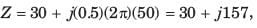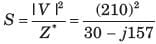Apparent power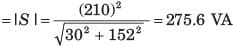QUESTION: 2

### In the circuit shown in fig. P1.7.35 power factor is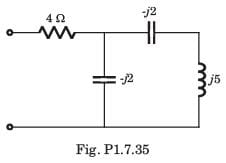Solution: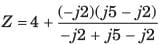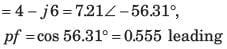QUESTION: 3

### The power factor seen by the voltage source is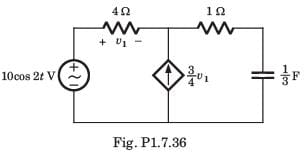Solution: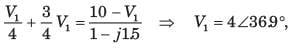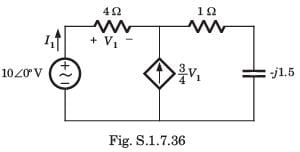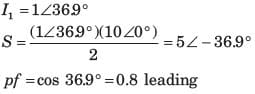QUESTION: 4

The average power supplied by the dependent source is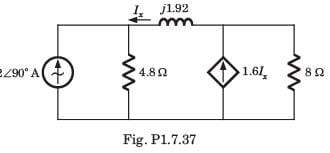Solution: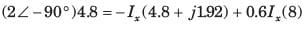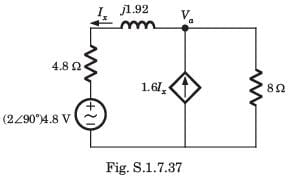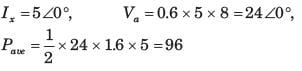QUESTION: 5

In the circuit of fig. P1.7.38 the maximum power absorbed by ZL is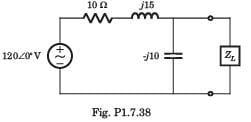Solution: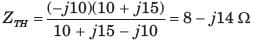QUESTION: 6

The value of the load impedance, that would absorbs the maximum average power is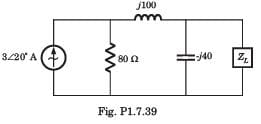Solution: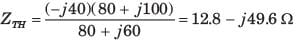QUESTION: 7

In a balanced Y–connected three phase generator Vab = 400 Vrms
Q. If phase sequence is abc then phase voltage Va, Vb,and Vc are respectively

Solution:

QUESTION: 8

In a balanced Y–connected three phase generator Vab = 400 Vrms
Q. If phase sequence is acb then phase voltage are

Solution:

For the acb sequence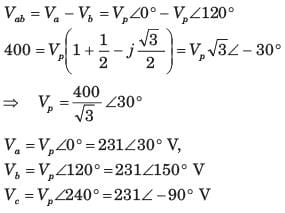QUESTION: 9

A balanced three-phase Y-connected load has one phase voltage Vc = 277∠450V. The phase sequence is abc. The line to line voltage VAB is

Solution: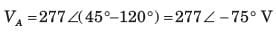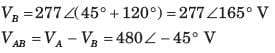QUESTION: 10

A three-phase circuit has two parallel balanced Δ loads, one of the 6Ω resistor and one of 12 Ω resistors. The magnitude of the total line current, when the line-to-line voltage is 480 Vrms ,is

Solution:

Z A = 6 ||12 = 4,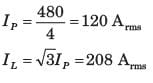Use Code STAYHOME200 and get INR 200 additional OFF Use Coupon Code